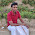## Friday, 1 March 2013

### C PROGRAM: TO MULTIPLY TWO NUMBERS WITHOUT USING ARITHMETIC OPERATOR *

CODING:
#include<conio.h>
#include<stdio.h>
void main()
{
int num1,num2,i,prod=0;
clrscr();
printf("Enter 1st number: ");
scanf("%d",&num1);
printf("Enter 2nd number: ");
scanf("%d",&num2);
for(i=1;i<=num2;i++)
{
prod+=num1;
}
printf("\n\n\nProduct of %d and %d is %d",num1,num2,prod);
getch();
}CODINGOUTPUT

For any query or suggestion please comment below...

#### 4 comments:

1.A much better method using bit manipulation(IN C++)

#include
using namespace std;
int main()
{
int a,b,c,i;
cin>>a>>b;
if(a>=1,i++)
{
if(b&1)c+=(a<<i);
}
cout<<c;
return 0;
}

2.Yes ok.But you are used + operator.So we can use sum+=i

3.it doesnt work because when we enter negative integer it counts as 0

4.This method is suitable for not all values, input 2,-3
Getting output is 0.
So how to solve this..